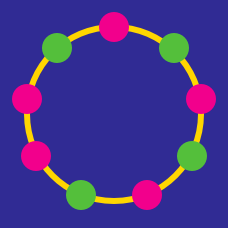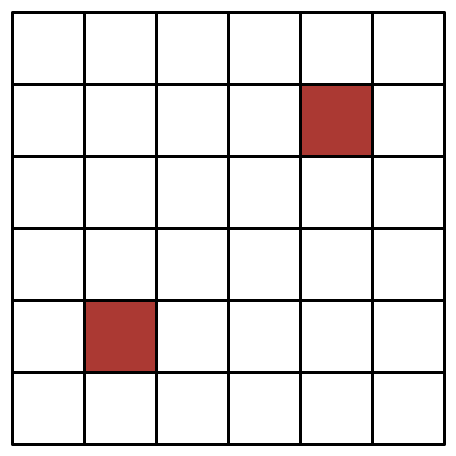Probability

# Discrete Mathematics Warmups: Level 5 ChallengesWhat is the probability (to 3 decimal places) that a point $$P$$ picked uniformly at random inside an equilateral triangle, is closer to the centroid than to its sides?

Consider the set $$\mathbb{S} =\{B,R,I,L,A,N,T\}$$. How many ways are there for us to partition the set into any number of non empty disjoint subsets whose Union is $$\mathbb{S}$$?.

Suppose two real numbers $$x$$ and $$y$$ are chosen, uniformly and at random, from the open interval $$(0,1)$$. Let $$P$$ be the probability that the integer closest to $$\dfrac{x}{y}$$ is odd.

Find $$\lceil 10000P \rceil$$.

Let $$a_0, a_1, \cdots, a_7$$ be any $$8$$ distinct integers. Let $$P$$ be the product of their pairwise differences, that is:

$P = \prod _ {i < j} {(a_i - a_j)}$

What is the greatest integer which always divides $$P?$$In the above image, how many rectangles are there which do not include any red squares?

×